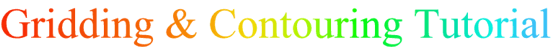XYZ Data Gridding Methods Size of Input Data Set Grid Maps Image Maps Shaded Relief Maps Vector Maps Wireframe Maps Adding Drawing Objects / Symbols The Importance of an Object Manager Variogram Modeling Conclusion### Vector Maps

A vector map shows direction and magnitude of data at points on a map. For example, consider a grid containing elevation information. If water were poured over the surface, the direction arrows would point in the direction water flows - from high elevation to low elevation. Magnitude is indicated by arrow length. In the water flow example, the steeper slopes would have longer arrows.

Information used to create vector maps can be contained in one grid  or in multiple grids..

In "one-grid" vector maps the two components of the vector map, direction and magnitude, are automatically generated from a single grid by computing the gradient of the surface. At any given grid node on the map, the arrow points in the direction of the steepest descent ("downhill"). The direction of the arrow changes from grid node to grid node depending on the topography surrounding the grid node. The magnitude of the arrow changes depending on the steepness of the descent.

In more advanced software packages such as , the two components of a vector map -    magnitude and direction  - can be created from two grids (or one grid if preferred). In addition, allows you to use either Cartesian or polar data with two-grid vector maps. (Note: with two-grid vector maps, both grids must have the same grid line geometry)

Two-grid vector maps use two separate grid files to determine the vector direction and magnitude. With Cartesian data, one grid consists of X component data and the other grid consists of Y component data. With polar data, one grid consists of angle information and the other grid contains length information. The data from the two grids are combined to produce vector direction and magnitude.

Vector maps created with polar data require that one grid contains angle (direction) data and the other contains length (magnitude) data. When creating vector maps with polar data you need to be aware that magnitude values cannot be negative. Thus, if you have chosen a gridding technique that displays trends in the data, such as Kriging, you may end up with negative values in your grid even if the original data are all positive. Regridding the magnitude data and decreasing the grid spacing is one way to make the grid honor the data better. However, if you regrid the magnitude data you must also remember to regrid the angle data using the same grid line geometry. If you do not want to change the grid line geometry of the grids or if decreasing the grid lines is not completely eliminating your negative (trending) data, then you may need to set all negative values equal to zero manually. A good software package such as has a "grid math" option which will allow you to do this at the click of a button.

Alternatively, use a gridding method that does not trend the data as much (eg. Triangulation with Linear Interpolation or Natural Neighbor).

There are various ways to scale vectors displayed on vector maps. For example, you can scale the symbols between the minimum and maximum data values linearly, logarithmically, or by square root. Linear scaling normally provides a better visual representation of symbols that are scaled in one dimension (such as arrows with varying shaft length). However, when scaling the arrows in two dimensions (symbol width and shaft length), Square Root or Logarithmic scaling often displays the arrows more effectively. A comprehensive software package such as offers a choice of ways to scale vectors. It also allows color scaling of the vectors.